## Example Questions

← Previous 1 3 4

### Example Question #1 : How To Find The Equation Of A Curve

Solve the equation for x and y.

x² + y = 31

x + y = 11

x = 13, 7

y = 8, –6

x = 6, 15

y = 5, –4

x = 8, –6

y = 13, 7

x = 5, –4

y = 6, 15

x = 5, –4

y = 6, 15

Explanation:

Solving the equation follows the same system as the first problem. However since x is squared in this problem we will have two possible solutions for each unknown. Again substitute y=11-x and solve from there. Hence, x2+11-x=31. So x2-x=20. 5 squared is 25, minus 5 is 20. Now we know 5 is one of our solutions. Then we must solve for the second solution which is -4. -4 squared is 16 and 16 –(-4) is 20. The last step is to solve for y for the two possible solutions of x. We get 15 and 6. The graph below illustrates to solutions.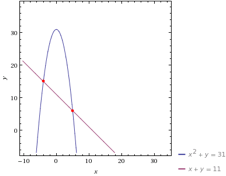### Example Question #1 : X And Y Intercept

Solve the equation for x and y.

x² – y = 96

x + y = 14

x = 5, –14

y = 15, 8

x = 25, 4

y = 10, –11

x = 15, 8

y = 5, –14

x = 10, –11

y = 25, 4

x = 10, –11

y = 25, 4

Explanation:

This problem is very similar to number 2. Derive y=14-x and solve from there. The graph below illustrates the solution.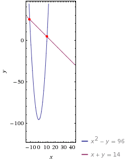### Example Question #3 : How To Find The Equation Of A Curve

Solve the equation for x and y.

5x² + y = 20

x² + 2y = 10

No solution

x = √10/3, –√10/3

y = 10/3

x = 14, 5

= 4, 6

x = √4/5, 7

= √3/10, 4

x = √10/3, –√10/3

y = 10/3

Explanation:

The problem involves the same method used for the rest of the practice set. However since the x is squared we will have multiple solutions. Solve this one in the same way as number 2. However be careful to notice that the y value is the same for both x values. The graph below illustrates the solution.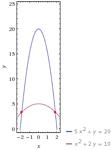### Example Question #4 : How To Find The Equation Of A Curve

Solve the equation for x and y.

x² + y = 60

x – y = 50

x = 40, 61

y = 11, –10

x = 11, –10

y = 40, 61

x = 10, –11

y = –40, –61

x = –40, –61

y = 10, –11

x = 10, –11

y = –40, –61

Explanation:

This is a system of equations problem with an x squared, to be solved just like the rest of the problem set. Two solutions are required due to the x2. The graph below illustrates those solutions.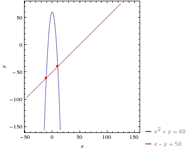### Example Question #5 : How To Find The Equation Of A Curve

A line passes through the points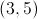and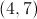. What is the equation for the line?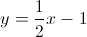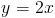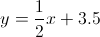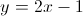Explanation:

First we will calculate the slope as follows: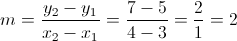And our equation for a line is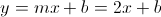Now we need to calculate b. We can pick either of the points given and solve for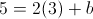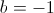Our equation for the line becomes### Example Question #1 : How To Find X Or Y Intercept

If the equation of a line is 4y – x = 48, at what point does that line cross the x-axis?

(–48,0)

(0,12)

(48,0)

(0,–12)

(0,–48)

(–48,0)

Explanation:

When the equation crosses the x-axis, y = 0.  Plug 0 into the equation for y, and solve for x

4(0) – x = 48,  –x = 48,  x = –48

### Example Question #2 : How To Find X Or Y Intercept

The slope of a line is equal to -3/4.  If that line intersects the y-axis at (0,15), at what point does it intersect the x-axis?

60

-20

20

15

5

20

Explanation:

If the slope of the line m=-3/4, when y=15 and x=0, plug everything into the equation y=mx+b.

Solving for b:

15=(-3/4)*0 + b

b=15

y=-3/4x + 15

To get the x-axis intersect, plug in y=0 and solve for x.

0 = -3/4x + 15

3/4x = 15

3x = 15*4

x = 60/3 = 20

x=20

### Example Question #1 : How To Find X Or Y Intercept

If these three points are on a single line, what is the formula for the line?

(3,3)

(4,7)

(5,11)

y = 4x + 31

y = 4x - 9

y = 3x - 9

y = 5x + 11

y = 3x - 3

y = 4x - 9

Explanation:

Formula for a line: y = mx + b

First find slope from two of the points: (3,3) and (4,7)

m = slope = (y2 – y1) / x2 – x1) = (7-3) / (4-3) = 4 / 1 = 4

Solve for b by plugging m and one set of coordinates into the formula for a line:

y = mx + b

11 = 4 * 5 + b

11 = 20 + b

b = -9

y = 4x - 9

### Example Question #4 : How To Find X Or Y Intercept

The slope of a line is 5/8 and the x-intercept is 16.  Which of these points is on the line?

(16, 10)

(32,10)

(32,30)

(0,10)

(8,15)

(32,10)

Explanation:

y = mx + b

x intercept is 16 therefore one coordinate is (16,0)

0 = 5/8 * 16 + b

0 = 10 + b

b = -10

y = 5/8 x – 10

if x = 32

y = 5/8 * 32 – 10 = 20 – 10 = 10

Therefore (32,10)

### Example Question #5 : How To Find X Or Y Intercept

A line has the equation: x+y=1.

What is the y-intercept?

1

0.5

0

2

-1